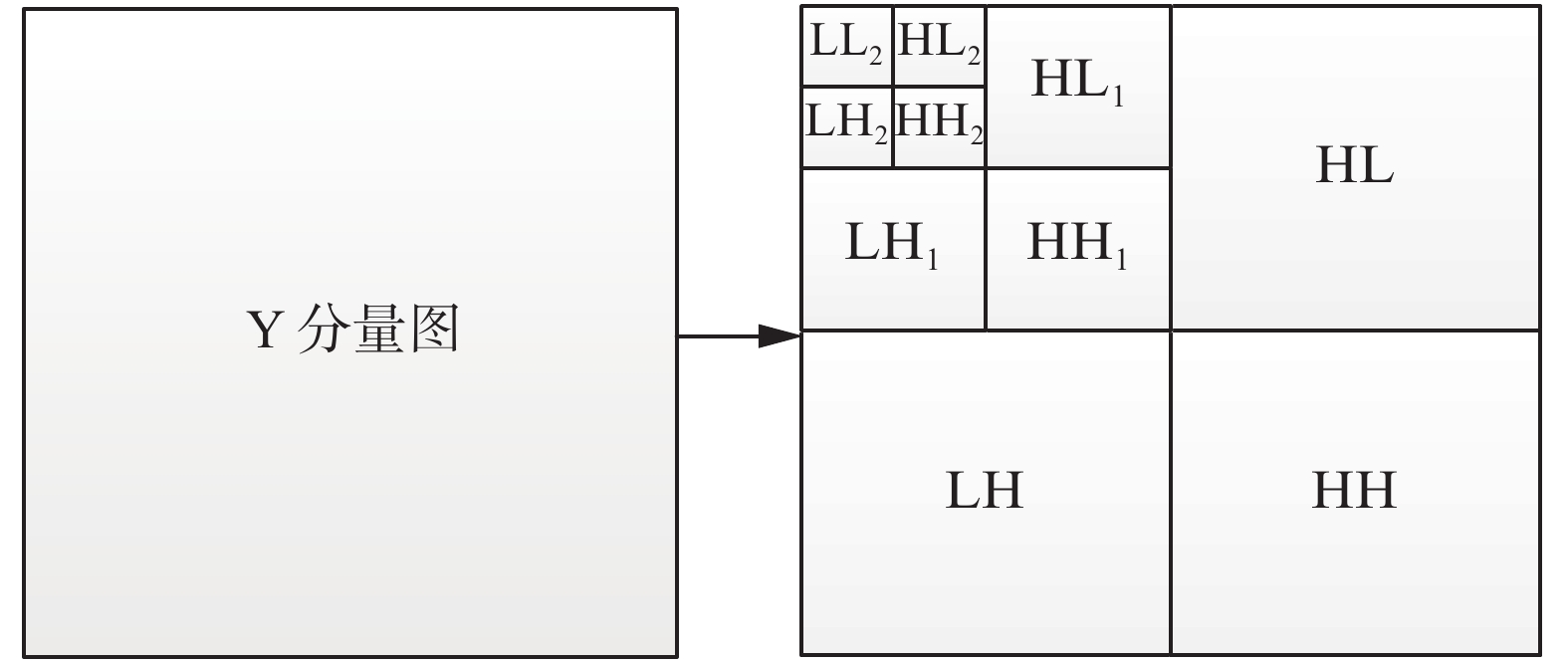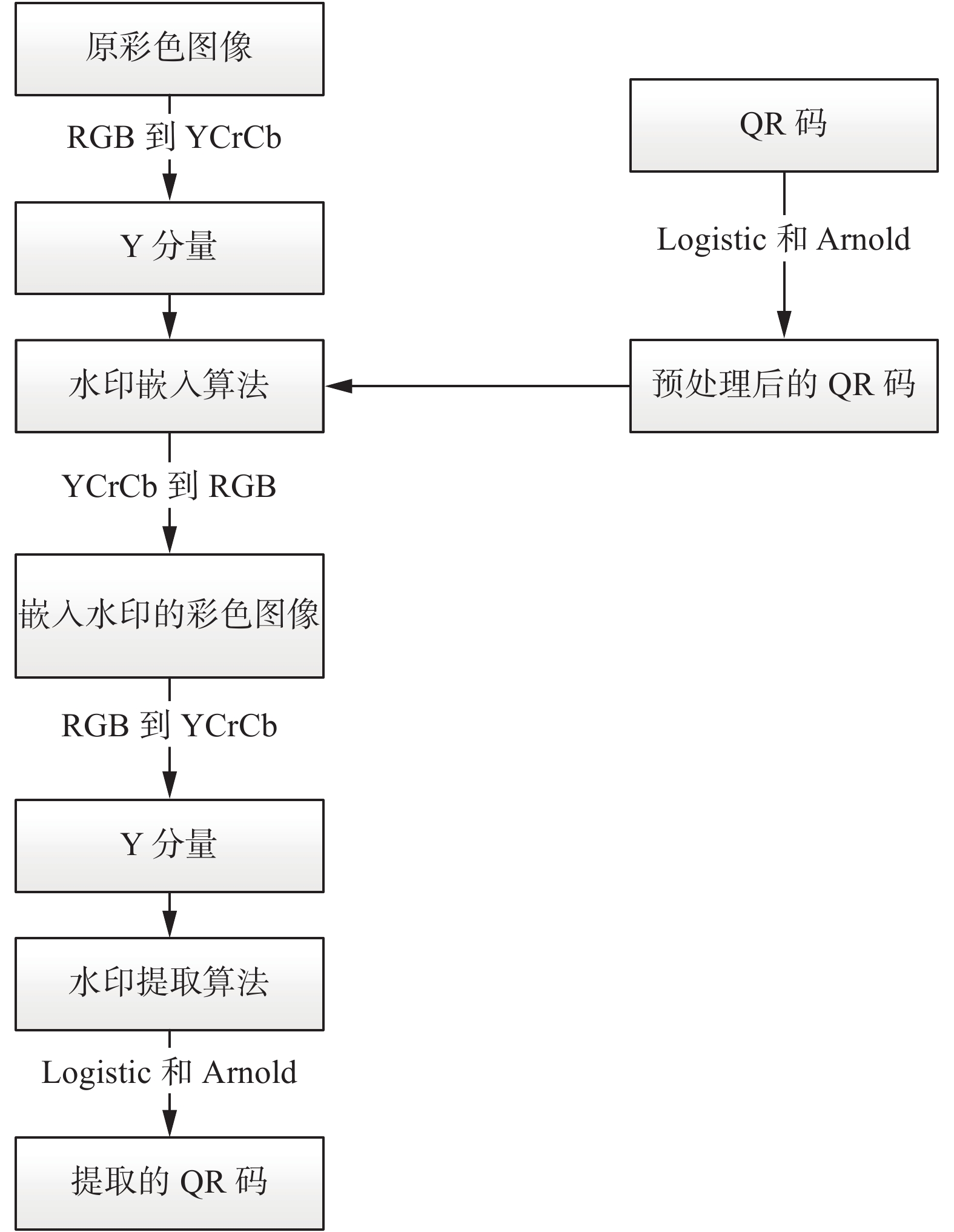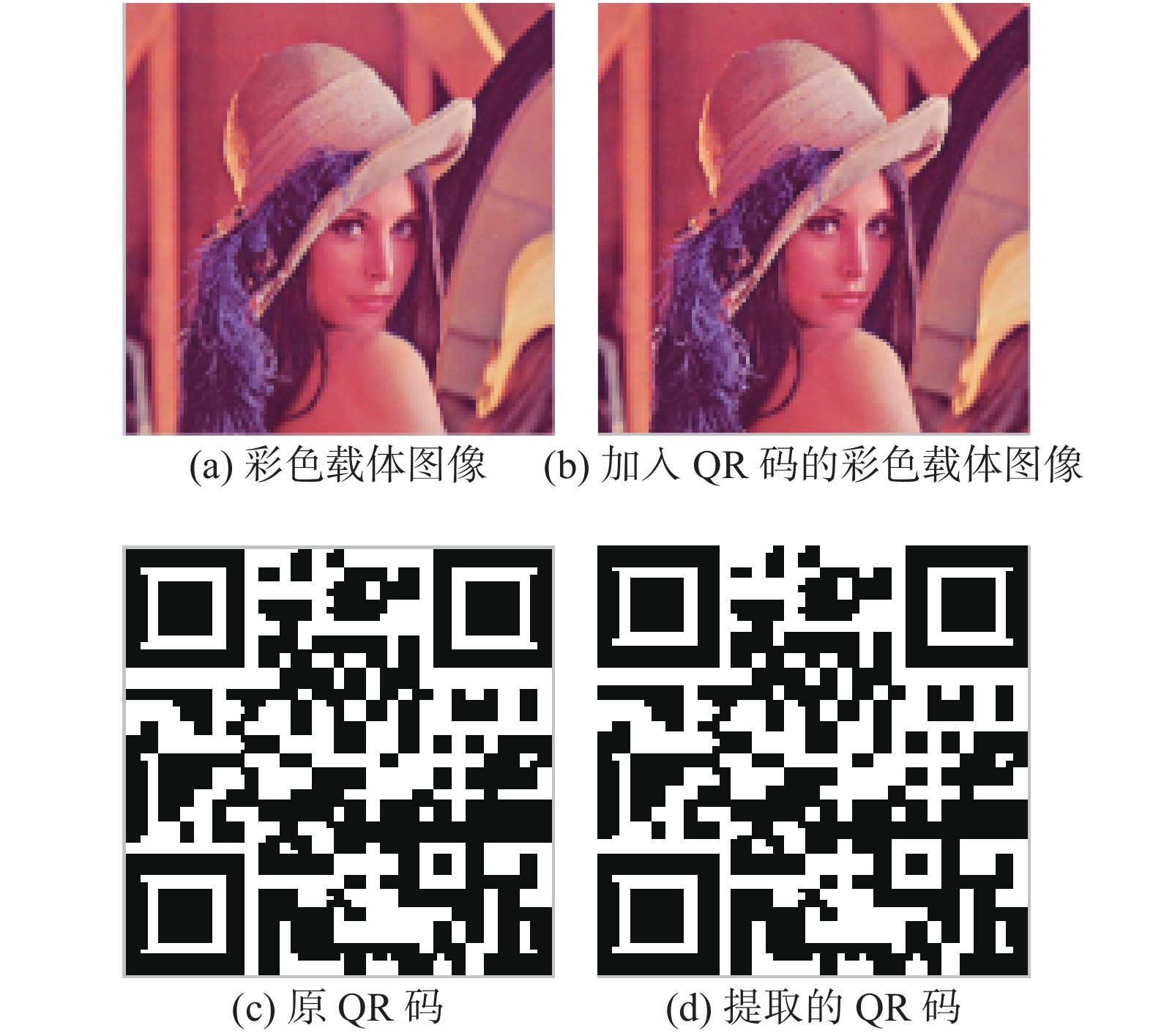﻿ 一种适用于QR码的彩色图像数字水印算法
«上一篇文章快速检索 高级检索

 智能系统学报2020, Vol. 15Issue (5): 949-955  DOI: 10.11992/tis.2019030160

### 引用本文SHEN Yanbing, YANG Yang, LI Zhu. Digital watermarking algorithm for color images on QR codes[J]. CAAI Transactions on Intelligent Systems, 2020, 15(5): 949-955. DOI: 10.11992/tis.201903016.### 文章历史

Digital watermarking algorithm for color images on QR codes
SHEN Yanbing , YANG Yang , LI ZhuSchool of Physics and Information Engineering, Shanxi Normal University, Linfen 041004, China
Abstract: Aiming at the poor robust performance of digital watermarking algorithms for color images, we propose a digital watermarking algorithm for color images applied to QR codes. A color carrier image is converted from RGB space to YCrCb space, in which the Y component is extracted and three-level discrete wavelet transform, discrete cosine transform, and singular value decomposition operations are gradually implemented to obtain the diagonal matrix; the QR code watermarking image implemented by a logistic chaotic map and an Arnold transform is successively embedded in the diagonal matrix through additive rules. The experimental results show that the peak signal-to-noise ratio (PSNR) value of the color image embedded with the QR code is 59.491 9. After the attacks of noises, compression, rotation, and contrast change, the PSNR and normalized correlation values are stably above 30 and 0.98, respectively. The extracted QR codes can be recognized by mobile phone scanning, which solves the poor anti-attack capability.
Key words: color image    QR code    Logistic chaotic mapping    Arnold transform    DWT    DCT    SVD    robustness

Zhang等提出对载体图像实施一级离散小波变换（discrete wavelet transform,DWT），选择其低频子图实施8×8分块，再进行离散余弦变换(discrete cosine transform, DCT)，并从4×4左上变换系数矩阵中提取组织新的矩阵实施奇异值分解(singular value decomposition,SVD)操作获得对角矩阵，最后在对角矩阵中嵌入水印。由实验数据可知，鲁棒性较强，但实验选取的载体图像非彩色图像，水印图像非QR码，因此实用性不足且嵌入量较小。徐江峰等提出对载体图像进行DWT操作，并对其低高频子带进行4×4分块DCT操作，将混沌加密后的QR码水印嵌入其中，实验选取的载体图像非彩色图像，实用性不强，在高斯噪声攻击和JPEG压缩攻击上，归一化相关系数（normalized correlation, NC）值略低。因此，鉴于以上问题，本文提出一种适用于QR码的彩色图像数字水印算法，该方案通过对QR码实施Logistic映射和Arnold变换来完成双重加密，用以增强水印的安全性能；对彩色载体图像从RGB空间转换到YCbCr空间，使用DWT、DCT和SVD技术在其亮度分量图中嵌入QR码，用来提高水印的嵌入量、不可见性和鲁棒性。

1 QR码水印的处理 1.1 Logistic映射

Logistic映射的定义为

 ${x_{k + 1}} = u{x_k}\left( {1 - {x_k}} \right)$

1.2 Arnold变换

Arnold变换实现加密的手段是将图像的像素点进行置乱。二维Arnold置乱为

 $\left[ \begin{array}{l} {x'} \\ {y'} \\ \end{array} \right] = \left[ \begin{array}{l} {\rm{1 1}} \\ {\rm{1 2}} \\ \end{array} \right]\left[ \begin{array}{l} x \\ y \\ \end{array} \right]\left( {{\rm{mod}}N} \right)$

2 彩色载体图像的处理

2.1 离散小波变换(DWT)Download: 图 1 小波变换分解 Fig. 1 Decomposition diagram of wavelet transform

2.2 离散余弦变换(DCT)

2.3 奇异值分解(SVD)

 ${{A}} = {{US}}{{{V}}^{\bf{T}}}$

3 水印的嵌入和提取Download: 图 2 水印算法的总框架 Fig. 2 The overall framework of the watermarking algorithm
3.1 水印的嵌入

1)对QR码先实施Logistic映射，后进行Arnold操作，获得双重加密后的QR码序列W

2)把彩色载体图像从RGB空间转换到YCbCr空间，提取其Y分量得到亮度分量图；

3)对亮度分量图实施3级DWT，获得低频子图LL，对LL实施DCT变换，即 ${\rm{dct}} = {\rm{dct}}2\left( {{\rm{LL}}} \right)$ ，得到变换后dct系数矩阵；

4)对dct系数矩阵实施奇异值分解，即 $\left[ {{{U}},{{S}},{{V}}} \right] =$ ${\rm{SVD}}\left( {{\rm{dct}}} \right)$ ，引入强度因子 $\alpha$ ，利用加性法则嵌入QR码信息，为

 ${{W}}a = {{S}} + \alpha {{W}}$

5)对 ${{W}}\alpha$ 矩阵实施奇异值分解，即 $\left[ {{{{U}}_1},{{{S}}_1},{{{V}}_1}} \right] =$ ${\rm{SVD}}\left( {{{W}}\alpha } \right)$ ，利用 ${{U}}{\text{、}}{{{S}}_1}{\text{、}}{{V}}$ 完成奇异值重构，即 ${\rm{dct}}1$ $= {{U}}{{{S}}_1}{{V}}$ 。最后实施DCT逆变换和3级小波逆变换，获得嵌入QR码的亮度分量图。

6)将嵌入QR码的亮度分量图从YCbCr空间转换到RGB空间，最终获得嵌入QR码的彩色载体图像。

3.2 水印的提取

1)将嵌入QR码的彩色载体图像从RGB空间转换到YCbCr空间，提取其Y分量获得亮度分量图；

2)对亮度分量图实施3级DWT，获得低频子图LL1，对LL1实施DCT变换，即 ${\rm{dct}}2 = {\rm{dct}}2\left( {{\rm{L}}{{\rm{L}}_1}} \right)$ ，得到dct系数矩阵；

3)对dct系数矩阵实施奇异值分解， $\left[ {{{{U}}_2},{{{S}}_2},{{{V}}_2}} \right]=$ ${\rm{SVD}}\left( {{\rm{dct}}2} \right)$ ，将 ${{{U}}_1},{{{S}}_2},{{{V}}_1}$ 进行逆奇异值变换，即 ${{SS}} = {{{U}}_1}{{{S}}_2}{{{V}}_1}$

4)利用 ${{S}},{{SS}},\alpha$ 提取双层加密后的QR码，即 ${{{W}}_b}$ $= \left( {{{SS}} - {{S}}} \right)/\alpha$ ，可以得到水印信息；

5)建立一个新的空矩阵Wc用于存放水印信息，当发现水印信息时，将其存入矩阵Wc中，因此，可得到加密后的QR码序列Wc

6)将QR码序列Wc实施解密操作，先实施Arnold变换，再实施Logistic映射，获得最终提取的QR码水印Ww

4 实验结果分析 4.1 水印评价标准

1) 该实验中，嵌入QR码的图像与未嵌入的原始图像之间的区别由峰值信噪比(peak signal-to-noise ratio, PSNR)来衡量。均方误差(mean-square error, MSE)、峰值信噪比PSNR的表达公式为

 ${\rm{MSE}} = \frac{1}{{MN}}\sum\limits_{i = 1}^n {\sum\limits_{j = 1}^N {\left[ {{{I}}'\left( {i,j} \right) - {{I}}\left( {i,j} \right)} \right]} }$ (1)
 ${\rm{PSNR}} = 10\log \frac{{{{255}^2} \times 3}}{{{E_{{\rm{MES}}}}\left( R \right) + {E_{{\rm{MES}}}}\left( G \right) + {E_{{\rm{MES}}}}\left( B \right)}}$ (2)

2)提取出的QR码与原QR码之间的相似性由归一化相关系数NC来衡量，为

 ${\rm{NC}} = \dfrac{{\displaystyle\sum\limits_{i = 1}^M {\displaystyle\sum\limits_{j = 1}^N {{{w}}(i,j){{w}}'(i,j)} } }}{{\displaystyle\sum\limits_{i = 1}^M {\displaystyle\sum\limits_{j = 1}^N {{{{w}}^2}(i,j)} } }}$ (3)

4.2 实验结果分析

4.2.1 未受攻击的实验结果Download: 图 3 未受攻击的实验结果 Fig. 3 Unattacked experimental results
4.2.2 受攻击的实验结果表 1 JPEG压缩测试结果 Tab.1 JPEG compression test results表 2 图像对比度和明暗变化测试结果 Tab.2 Test results of image contrast and brightness changes表 3 噪声测试结果 Tab.3 Noise test results表 4 剪切测试结果 Tab.4 Shear test results表 5 旋转测试结果 Tab.5 Rotation test results

1) JPEG压缩测试

2)图像对比度和明暗变化测试

3)噪声测试

4)剪切测试

5)旋转测试

4.2.3 与其他算法相比较的实验结果表 6 本文与文献的实验结果比较 Tab.6 Comparison of experimental results between this article and literature 
5 结束语

  王晓红, 孙业强. 基于QR分解的强鲁棒性双彩色盲水印算法[J]. 光电子·激光, 2017, 28(9): 1016-1024. WANG Xiaohong, SUN Yeqiang. Robust dual color blind watermarking algorithm based on QR decomposition[J]. Journal of optoelectronics·laser, 2017, 28(9): 1016-1024. (0)  王子煜, 孙刘杰, 李孟涛. 强鲁棒性QR码水印技术[J]. 包装工程, 2012, 33(15): 84-87. WANG Ziyu, SUN Liujie, LI Mengtao. QR code watermark technology with strong robustness[J]. Packaging engineering, 2012, 33(15): 84-87. (0)  ZHANG Zhi, WANG Chengyou, ZHOU Xiao. Image watermarking scheme based on Arnold transform and DWT-DCT-SVD[C]//Proceedings of the 2016 IEEE 13th International Conference on Signal Processing. Chengdu, China, 2016: 805−810. (0)  徐江峰, 张守强. 基于QR码的DWT-DCT数字水印算法[J]. 计算机应用研究, 2018, 35(5): 1540-1544. XU Jiangfeng, ZHANG Shouqiang. Algorithm of DWT-DCT digital watermarking based on QR code[J]. Application research of computers, 2018, 35(5): 1540-1544. DOI:10.3969/j.issn.1001-3695.2018.05.056 (0)  季诺然, 吕晓琪, 谷宇, 等. 基于QR码与混沌加密的Contourlet域彩色图像盲水印算法[J]. 包装工程, 2017, 38(15): 173-178. JI Nuoran, LYU Xiaoqi, GU Yu, et al. Blind watermarking algorithm for color image in contourlet domain based on QR code and chaotic encryption[J]. Packaging engineering, 2017, 38(15): 173-178. (0)  王晓红, 魏代海, 刘玄玄, 等. 一种彩色QR码嵌入彩色图像的数字水印技术[J]. 光电子·激光, 2016, 27(10): 1094-1100. WANG Xiaohong, WEI Daihai, LIU Xuanxuan, et al. Digital watermarking technique of color image based on color QR Code[J]. Journal of optoelectronics·laser, 2016, 27(10): 1094-1100. (0)  JANE O, ELBAŞI E, İLK H G. Hybrid non-blind watermarking based on DWT and SVD[J]. Journal of applied research and technology, 2014, 12(4): 750-761. DOI:10.1016/S1665-6423(14)70091-4 (0)  THIND D K, JINDAL S. A semi blind DWT-SVD video watermarking[J]. Procedia computer science, 2015, 46: 1661-1667. DOI:10.1016/j.procs.2015.02.104 (0)  王世辉, 王仪明, 武淑琴, 等. 萤火虫算法优化的小波域图像水印技术[J]. 微电子学与计算机, 2018, 35(5): 124-127, 132. WANG Shihui, WANG Yiming, WU Shuqin, et al. A watermarking method in wavelet domain optimized by firefly algorithm[J]. Microelectronics and computer, 2018, 35(5): 124-127, 132. (0)  孔玲君, 聂鹏. 一种基于DWT-DCT变换域的全息水印技术[J]. 光电子·激光, 2016, 27(2): 198-202. KONG Lingjun, NIE Peng. A holographic digital watermarking technology based on DWT-DCT transform domain[J]. Journal of optoelectronics·laser, 2016, 27(2): 198-202. (0)  刘丽, 周亚建, 张斌, 等. 基于DCT和SVD的QR码数字水印算法[J]. 红外与激光工程, 2013, 42(S2): 304-311. LIU Li, ZHOU Yajian, ZHANG Bin, et al. Digital watermarking method for QR code images based on DCT and SVD[J]. Infrared and laser engineering, 2013, 42(S2): 304-311. (0)  孙业强, 王晓红, 李肖赫. 基于QR码和DWT-SVD技术的双彩色盲水印算法[J]. 包装工程, 2017, 38(11): 193-198. SUN Yeqiang, WANG Xiaohong, LI Xiaohe. Double color blind watermarking algorithm based on QR Code and DWT-SVD[J]. Packaging engineering, 2017, 38(11): 193-198. (0)  ALI M, AHN C W, PANT M. A robust image watermarking technique using SVD and differential evolution in DCT domain[J]. Optik, 2014, 125(1): 428-434. DOI:10.1016/j.ijleo.2013.06.082 (0)  吴军, 孙像军, 李慧慧. 一种基于彩色图像的双重水印算法[J]. 电视技术, 2018, 42(2): 101-109, 114. WU Jun, SUN Xiangjun, LI Huihui. A dual watermarking algorithm based on color image[J]. Video engineering, 2018, 42(2): 101-109, 114. (0)  汤永利, 张亚萍, 高玉龙, 等. 基于DWT-SVD压缩量化的数字图像盲水印算法[J]. 重庆邮电大学学报(自然科学版), 2018, 30(2): 265-271. TANG Yongli, ZHANG Yaping, GAO Yulong, et al. Compression quantitative digital image blind watermarking algorithm based on DWT-SVD[J]. Journal of Chongqing University of Posts and Telecommunications (Natural Science Edition), 2018, 30(2): 265-271. (0)  肖振久, 郭冰莹, 李南, 等. 一种基于Slant变换和SVD的稳健性数字水印算法[J]. 计算机工程与科学, 2018, 40(10): 1772-1779. XIAO Zhenjiu, GUO Bingying, LI Nan, et al. A robust digital watermarking algorithm based on Slant transform and SVD[J]. Computer engineering and science, 2018, 40(10): 1772-1779. DOI:10.3969/j.issn.1007-130X.2018.10.008 (0)  陈青, 郭功勋. 一种基于模运算的小波系数调整水印算法[J]. 小型微型计算机系统, 2019, 40(2): 395-400. CHEN Qing, GUO Gongxun. Wavelet coefficient adjustment watermark algorithm based on modular arithmetic[J]. Journal of Chinese computer systems, 2019, 40(2): 395-400. DOI:10.3969/j.issn.1000-1220.2019.02.027 (0)  任克强, 梁亮亮, 喻玲娟. SVD与HVS相结合的小波域数字图像鲁棒水印[J]. 电子测量与仪器学报, 2017, 31(6): 869-875. REN Keqiang, LIANG Liangliang, YU Lingjuan. Robust digital image watermarking in wavelet domain based on SVD and HVS[J]. Journal of electronic measurement and instrumentation, 2017, 31(6): 869-875. (0)  姚军财. 基于小波变换和奇异值分解的图像水印算法研究[J]. 光学技术, 2017, 43(5): 439-444. YAO Juncai. Image watermark algorithm base on the discrete wavelet transform and singular value decomposition[J]. Optical technique, 2017, 43(5): 439-444. (0)  曲长波, 于智龙, 李栋栋. 基于分块FRIT-SVD的鲁棒零水印算法[J]. 计算机工程与科学, 2018, 40(6): 1005-1016. QU Changbo, YU Zhilong, LI dongdong. A robust zero-watermarking algorithm based on the block FRIT-SVD[J]. Computer engineering and science, 2018, 40(6): 1005-1016. DOI:10.3969/j.issn.1007-130X.2018.06.007 (0)